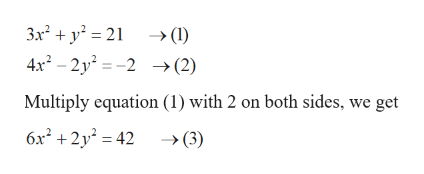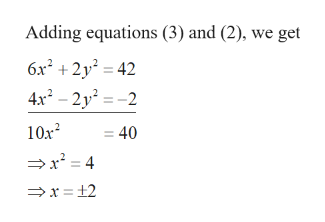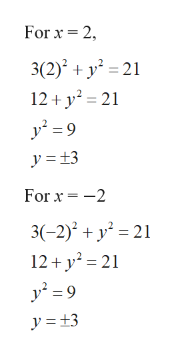# For Exercise, solve the system by using the addition method. 3x2 +y2 =214x2 -2y2 =-2

Question
8 views

For Exercise, solve the system by using the addition method.

3x+y=21

4x-2y=-2

check_circle

Step 1

We have givenhelp_outlineImage Transcriptionclose3x² + y² = 21 → (1) 4x² – 2y² = -2 →(2) Multiply equation (1) with 2 on both sides, we get 6x² +2y² = 42 → (3) fullscreen
Step 2

Solvehelp_outlineImage TranscriptioncloseAdding equations (3) and (2), we get 6x² + 2y? = 42 4x? – 2y² = -2 10x? ,2 = 40 →x = 4 =x = +2 fullscreen
Step 3

Substitute the values of x in equatio...help_outlineImage TranscriptioncloseFor x = 2, 3(2)² + y² = 21 12 + y? = 21 y² = 9 y =13 For x = -2 3(-2)² + y² = 21 12+ y² = 21 y = 9 y = +3 fullscreen

### Want to see the full answer?

See Solution

#### Want to see this answer and more?

Solutions are written by subject experts who are available 24/7. Questions are typically answered within 1 hour.*

See Solution
*Response times may vary by subject and question.
Tagged in

### Algebra